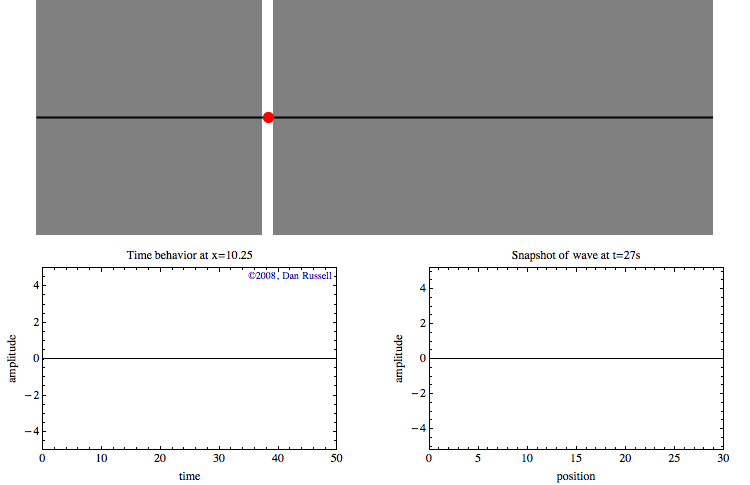# Science Tutorial: Wavelength

A wave has a repeating pattern. One complete repetition of the pattern is a cycle.  The time to complete a cycle is the period. The distance that a sound wave travels in one period is called the wavelength.  Wavelength is related to the speed at which sound travels and can be calculated by dividing the speed of sound by the frequency of the sound:

Wavelength = Speed of sound / Frequency of sound

It is important to remember that a sound wave can be thought of in relation to time and space (distance).  The animation below shows a wave pulse traveling from left to right. As the wave passes a location, a red dot highlights the time and space behavior at that specific location in the wave’s motion.

The graph on the lower left shows the time history of the movement of this red dot as the wave passes by. This graph represents the wave as a function of time for a specific location. When the leading edge of the wave reaches the specified location (with the red dot), the position of the dot moves up and down with time as the wave passes. After the wave pulse has passed through, the dot stops moving.

The graph on the lower right represents a snap shot of the wave position at each second after the waves travels for a duration of 27 seconds. This graph represents the wave as a function of position at a specific time.A wave is a disturbance that travels from one location to another, and is described by a wave function that is a function of both space and time. Image used with permission, copyright: Dan Russell, Grad. Prog. Acoustics, Penn State, https://www.acs.psu.edu/drussell/Demos/waves/wavemotion.html.

A high-frequency sound has a shorter wavelength than a low-frequency sound. Using 1,500 meters per second for the approximate speed of sound in seawater we find the following relationships between frequency and wavelength, calculated using the equation given above:

 Frequency (hertz, or cycles per second) Wavelength (meters) 100 15 1,000 1.5 10,000 0.15